# 《编程珠玑（续）（修订版）》—第2章2.3节拓扑排序

2.3　拓扑排序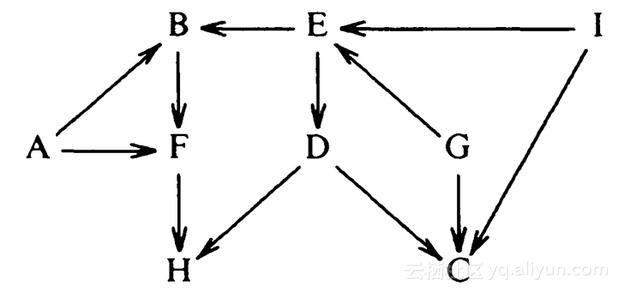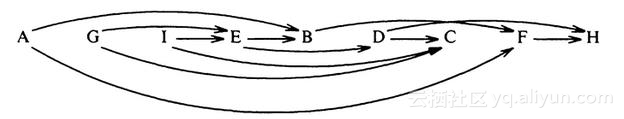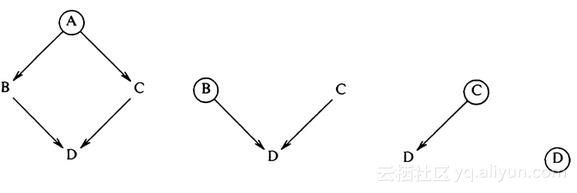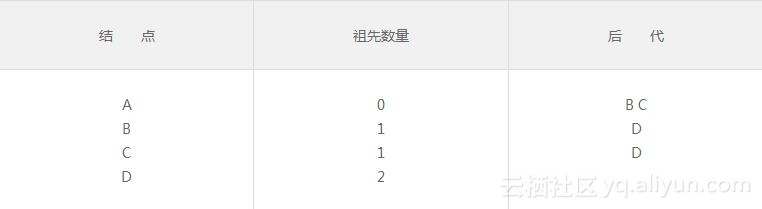as each (pred, succ) pair is read
increment pred count of succ
append succ to successors of pred
at the end of the input file
initialize queue to empty
for each node i
if pred count of i is zero then append i to queue
while queue isn't empty do
delete t from front of queue; print t
for each successor s of t
decrement pred count of s
if that goes to zero then append x to queue
if some nodes were not output then report a cycle


Awk程序使用一个索引范围是1..n的数组来实现队列。整型变量qlo是队列首元素的索引，qhi是尾元素的索引。后代集合由两个数组实现。如果A有后代B、C、D，那么下面的关系成立：

succct["A"] == 3
succlist["A",　 "1"] == "B"
succlist["A",　 "2"] == "C"
succlist["A",　 "3"] == "D"


{ ++predct[$2] _#_ record nodes in predct, predct[$1] = predct[$1] _#_ even those with no preds succlist[$1, ++succcnt[$1]] =$2
}
END  { qlo = 1
for (i in predct)  {
n++;　if (predct[i] == 0) q[++qhi] = i
}
while (qlo <= qhi)  {
t = q[qlo++]; print t
for (i = 1; i <= succcnt[t]; i++)  {
s = succlist[t, i]
if (--predct[s] == 0) q[++qhi] = s
}
}
if (qhi != n) print "tsort error: cycle in input"
}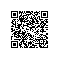使用钉钉扫一扫加入圈子
+ 订阅